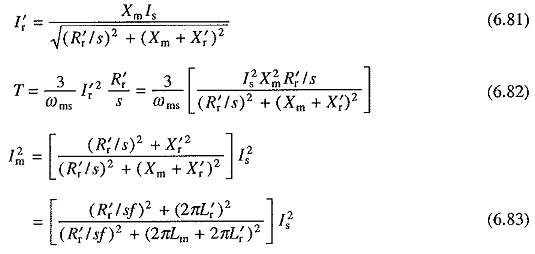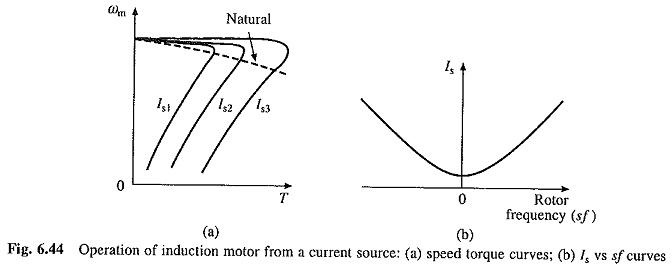## Variable Frequency Control From Current Source:

Control of induction motor employing variable frequency voltage sources was considered in previous section. This section considers motor control by Variable Frequency Control From Current Source (VFCS). An equivalent circuit for motor fed from a current source is obtained when voltage source V is replaced by a current source Is in Fig. 6.1(a). NowMotor speed-torque curves for various values of Is and natural speed-torque curve, which corresponds to the operation at rated constant flux, are shown in Fig. 6.44(a). For a given Is, operation of motor above the natural characteristic takes place for a flux higher than rated and below it at lower than rated. Since rated flux operation is preferred due to reasons explained already, the natural characteristic is locus of preferred, operating points. From Eq. (6.83), one can obtain a relationship between Is and rotor frequency (sf) for rated Im (or rated flux). This relationship, which is independent of frequency, is shown in Fig. 6.44(b). Drive is operated such that relationship of Fig. 6.44(b) is maintained between stator current Is and rotor frequency (sf), when frequency is changed to control the speed.When operating at a constant flux, the operating points are located mostly on the part of speed torque curve, which gives unstable operation with most loads (Fig. 6.44(a)). Hence, closed loop control is mandatory. Since motor is constraint to operate at constant flux, its steady-state behavior is identical to that with VFVS. Thus at a given slip speed (or rotor frequency), the motor draws a constant current and develops a constant torque at all frequency, as explained already.

The motor, therefore, operates in constant torque mode from zero to base speed. At base speed, either rated machine voltage is reached or VFCS voltage saturates. In either case machine operates at a constant terminal voltage above base speed, providing constant power mode. Variable frequency current supply is provided by a Variable Frequency Control From Current Source inverter.

Scroll to Top